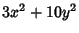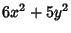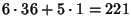## Composition

The combination of two Functions to form a single new Function. The composition of two functionsandis denotedand is defined bywhenandare both functions of.

An operation called composition is also defined on Binary Quadratic Forms. For two numbers represented by two forms, the product can then be represented by the composition. For example, the composition of the formsandis given by, and in this case, the product of 17 and 13 would be represented as (). There are several algorithms for computing binary quadratic form composition, which is the basis for some factoring methods.